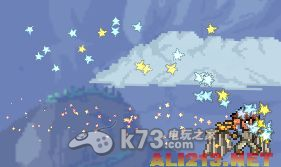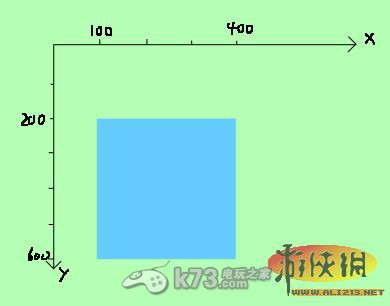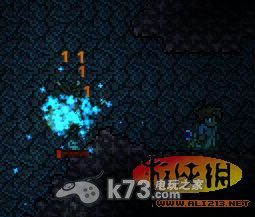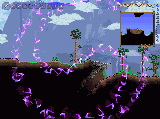# ::Android::台灣網

Android遊戲,軟體資訊,android手機ROOT

## 泰拉瑞拉飾品制作方法

《泰拉瑞拉》的飾品制作方法其實還是有一些麻煩的，玩傢們初上手制作可能會遇到一些困難，不過我想這些都不是玩傢們想要制作飾品的阻礙，希望以下的飾品的制作方法，可以讓玩傢們在這款遊戲中可以獲得更多的樂趣。

【基礎篇：飾品創建】

png就不多說瞭，圖片，不要找太大的就行瞭，反例請參見ctx的just a simple boss裡的BOSS召喚物——骷髏下巴，因為直接用瞭骷髏下巴的圖，太大，撿起來放在物品欄的時候縮得不成樣子

{

“code”: “ctxmove”,——名字，要和json的文件名一致

“displayName”: “CTXs Movement”,——顯示名稱，最好區分大小寫以顯得很正式

“size”: [28,14],——尺寸，兩個數字分別對應png圖像的長和寬

“value”: [0,0,0,0],——價值，四個數字分別對應鉑、金、銀、銅幣的數量（註意這裡是買入價值，賣出時會有折扣，使用打折卡買入也會有折扣）

“rare”: 9,——稀有度，⑨就是最稀有的天藍瞭好像

“toolTip”: “I hate json.”,——第一行道具說明（想加第二行就是toolTip2）

“accessory”:true,——這句是重點，它決定瞭這個道具可以被塞進飾品欄

“recipes”: [——合成表

{

“items”: [“0 Wood”],——用0個木頭

“creates”: 1——合成1個飾品

}

]——這裡的recipe明顯不合格好吧！連tile也沒有——嘛，這不是重點

}

【進階篇：cs創建】

using System;

using TAPI;

using Microsoft.Xna.Framework.Graphics;

namespace TAPI.【MOD名稱】{

public class 【道具名稱】:ModItem{

public 【道具名稱】(ModBase modbase,Item i):base(modbase,i){}

public override void Effects(Player p){

//等會就是在這裡插入飾品信息滴～

}

}

p.statManaMax=200;同理，玩傢最大魔法值飆到200

p.statManaMax2=+200;這個就像一般的法師飾品一樣暫時增加瞭玩傢的最大魔法，卸掉飾品就會還原（而前兩個不會）

p.wings = 12;給玩傢戴上蒸汽朋克翅膀

p.statDefense+=233;玩傢防禦增加233

p.lifeRegen+=233;玩傢回血速度增加233（再註：這裡的回血速度最大也隻能增加到每秒一點，是TR原版回血機制的問題）

p.statDefense+=2333333333;神馬的就不用我說瞭吧～改數字而已，大傢自便，不要讓自己失去遊戲樂趣就可以瞭

p.statLife++;

——什麼？還是太弱瞭？你手殘所以每秒60血根本不夠？

p.longInvince = true;

p.lavaImmune = true;

p.fireWalk = true;

p.noFallDmg = true;

——什麼？你還想站擼霜月？

p.statLife=p.statLifeMax;和p.statMana=p.p.statManaMax2;

——什麼？你還要白天去同時站擼骷髏王、地牢守護者和機械骷髏王？

【喪病篇：鬼畜cs進階】

……剛才送快遞的LZW小哥告訴我，不能把這個說出來，不然會(ta)極(hui)大(zai)地(jin)影(wan)響(lai)遊(gang)戲(le)平(wo)衡，所以就隻能給一點提示瞭：給玩傢強行上無敵～

1.時裝

p.body=65;

p.legs=54;

p.body=66;

p.legs=55;

2.套裝效果

p.thorns = true;

p.turtleThorns = true;

p.ghostHeal=true;

p.lifeSteal=233f;——瞬間還原至舊版幽靈套的吸血頻率我會說？

3.視覺特效

for (int i=0;i<9;i++){

int m = Dust.NewDust(p.position, p.width, p.height, 6);

Main.dust[m].noGravity = true;

Main.dust[m].position += p.velocity * Main.rand.Next(-50, 51) * 0.01f;

}

Gore.NewGore(p.position+new Vector2(Main.rand.Next(p.width),Main.rand.Next(p.height)), p.velocity,Main.rand.Next(16,18));

【DEBUG-查錯階段】using System;

using TAPI;

using Microsoft.Xna.Framework;

namespace TAPI.【MOD名稱】{

public class 【道具名稱】:ModItem{

public 【道具名稱】(ModBase modbase,Item i):base(modbase,i){}

public override void Effects(Player p){

//等會就是在這裡插入飾品信息滴～

}

}

1.Rectangle(組詞法：rect是“四”，angle是“角”，四個墻角即為矩形～)

TR裡聲明一個矩形的方法是使用new方法組，也就是new Rectangle(坐標X,坐標Y,寬,高)2.Rectangle.Intersects(Rectangle r1,Rectangle r2)

intersects方法組，隸屬於xna特有的數學系（重申一遍前面cs格式的.Graphics一定要刪掉！），用來判定兩個矩形是否相交，輸出邏輯值（真或假）

p.s.判定是否在范圍內時好像比直接計算距離略快一點，缺點就是判定是方的不好看

……嘛，戰鬥時誰還管好不好看呢？

3.foreach(類型 代號 in 組)

·BUFF類型就是想要加的BUFF的編號，比如燃燒是24，詛咒燃燒是39，霜火燃燒是44，等等

·BUFF持續時間是按幀算的，60幀=1秒（0處理落率），如果這裡寫60就是一秒，寫3600是一分鐘，寫23333333就是約4.50102874天，依據自己需求來決定時間吧

5.if(條件)

5.2.else

“否則”是“如果”的好姬友，用於在if的條件不成立時執行後面的語句

5.2.1.多層判定

if(條件1){代碼段1}

else if(條件2){代碼段2}

else if(條件3){代碼段3}

else……

……

……else{都不滿足時的代碼段}

5.2.邏輯值的運算

5.2.1.幾個邏輯運算的法則

1.!!a就是a

2.!(a&&b)就是(!a)||(!b)

3.!(a||b)就是(!a)&&(!b)

if(b||c)m++;

if(d&&e)m++;

if(f)m++;

M=(!(a||b))&&(m>=2)

6.反擊時刻！

6.1.思路（伸手黨可以跳過這一段直接復制後方的成品，感興趣的還是看一下吧～）

NPC所在的矩形是new Rectangle((int)n.position.X,(int)n.position.Y,n.width,n.height)

if(n.active&&!n.friendly&&new Rectangle((int)n.position.X,(int)n.position.Y,n.width,n.height).Intersects(new Rectangle((int)p.position.X-300,(int)p.position.Y-300,p.width+600,p.height+600)))

6.2.成品代碼：

foreach(NPC n in Main.npc){

if(n.active&&!n.friendly&&new Rectangle((int)n.position.X,(int)n.position.Y,n.width,n.height).Intersects(new Rectangle((int)p.position.X-300,(int)p.position.Y-300,p.width+600,p.height+600))){

}

}

6.3.效果圖：BURN BABY,BURN!

①proj對應的組是Main.projectile，proj的類型是Projectile

②proj和NPC一樣有位置和寬高數據，位置可以被任意操作

③敵對NPC特征是friendly邏輯值為假，敵對proj特征是hostile邏輯值為真

【獵奇篇：讀取操作】

1.讀取玩傢行動

controlLeft;控制向左

controlRight;控制向右

controlUp;控制向上

controlDown;控制向下

controlJump;控制跳

controlHook;控制鉤子

if (p.controlLeft)

{

if (p.velocity.X > 0) p.velocity.X *= 0.95f;

else if (p.velocity.X > -30) p.velocity.X -= p.velocity.Y == 0 ? 0.5f : 1f;

}

if (p.controlRight)

{

if (p.velocity.X < 0) p.velocity.X *= 0.95f;

else if (p.velocity.X < 30) p.velocity.X += p.velocity.Y == 0 ? 0.5f : 1f;

}

if (p.controlUp)

{

p.controlJump = true;

if (p.velocity.Y > 0) p.velocity.Y *= 0.95f;

else if (p.velocity.Y > -30) p.velocity.Y -= 1f;

}

2.讀取鼠標狀態

3.讀取鍵盤狀態

Main.keyState.IsKeyDown(Microsoft.Xna.Framework.Input.Keys.【按鍵代號】)

【獵奇+篇：輔助火力】

1.Projectile.NewProjectile(坐標X,坐標Y,速度X,速度Y,種類,傷害,擊退,所屬玩傢,ai0,ai1)

①三角函數天才，或者平面向量萬年掛科，請前往條目2

②平面向量學霸或者痛恨三角函數，請前往條目3

1.1.先確定自己和鼠標的位置吧～

Vector2 pc=p.position+new Vector2(p.width,p.height)/2;

Vector2 mc=Main.screenPosition+new Vector2(Main.mouseX,Main.mouseY);

2.Math.Atan2(對邊,斜邊)

float r=(float)Math.Atan2(mc.Y-pc.Y,mc.X-pc.X);

(這個函數是反正切，又不是反正切，因為它的對邊和斜邊隨便哪個取0都是可以輸出結果的；而且它的值域是2π，也就是一圈！)

3.Vector2.Distance(向量1,向量2)

Q:沒事求向量長度幹啥？

A:向量除以長度等於單位向量

Vector2 pc=p.position+new Vector2(p.width,p.height)/2;

Vector2 mc=Main.screenPosition+new Vector2(Main.mouseX,Main.mouseY);

Vector2 v=(mc-pc)/Vector2.Distance(mc,pc);

Projectile.NewProjectile(pc.X,pc.Y,v.X*6,v.Y*6,255,50,0,p.whoAmI);

【獵奇+篇：控制法】

0.特別說明

1.單發

using System;

using TAPI;

using Microsoft.Xna.Framework;

namespace TAPI.【MOD名稱】{

public class 【道具名稱】:ModItem{

public 【道具名稱】(ModBase modbase,Item i):base(modbase,i){}

bool oldF=false;

public override void Effects(Player p){

if(p.whoAmI==Main.myPlayer&&!oldF&&Main.keyState.IsKeyDown(Microsoft.Xna.Framework.Input.Keys.F)){

//這裡面就是寫前面發射子彈代碼的地方

}

oldF=Main.keyState.IsKeyDown(Microsoft.Xna.Framework.Input.Keys.F;

}

}

2.連發

using System;

using TAPI;

using Microsoft.Xna.Framework;

namespace TAPI.【MOD名稱】{

public class 【道具名稱】:ModItem{

public 【道具名稱】(ModBase modbase,Item i):base(modbase,i){}

int timer=0;

public override void Effects(Player p){

if(timer>0)timer–;

else{

if(p.whoAmI==Main.myPlayer&&Main.keyState.IsKeyDown(Microsoft.Xna.Framework.Input.Keys.F)){

timer=10;

//這裡面就是寫前面發射子彈代碼的地方

}

}

}

}

3.自動

using System;

using TAPI;

using Microsoft.Xna.Framework;

namespace TAPI.ctx{

public class ctxbullet : ModItem{

int timer = 0;

float r = 0;

float rv = 0;

float ra = 0.001f;

bool barrage = false;

public ctxbullet(ModBase modbase, Item i) : base(modbase, i) { }

public override void Effects(Player p){

rv += ra;

if (rv > 3) rv -= (float)Math.PI * 2f;

r += rv;

if (r > 3) r -= (float)Math.PI * 2f;

timer++;

if (timer > 3) timer -= 3;

if (barrage){

if (p.statMana < 3 || p.whoAmI != Main.myPlayer){

barrage = false;

goto outOfMana;

}

else p.statMana -= 3;

if (timer % 3 == 0){

Vector2 pc = p.position + new Vector2(p.width, p.height) / 2;

Vector2 v = new Vector2((float)Math.Cos(r), (float)Math.Sin(r));

Projectile.NewProjectile(pc.X, pc.Y, v.X * 20f, v.Y * 20f, 274, 100, 0, p.whoAmI);

Projectile.NewProjectile(pc.X, pc.Y, -v.X * 20f, -v.Y * 20f, 274, 100, 0, p.whoAmI);

}

}

outOfMana:

if (Main.keyState.IsKeyDown(Microsoft.Xna.Framework.Input.Keys.F)) barrage = !barrage;

}

}

}（所以說彈幕可以培養數學和想象力=w=）